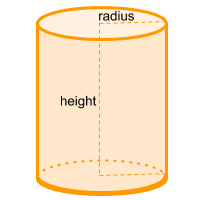Home » Non-polyhedra » Cylinder

# Volume of a CylinderVolume =

## Volume calculator for a cylinder

Enter the radius and height of the cylinder to get the volume automatically.

## Description, how many faces, edges and vertices are there in a cylinder

The cylinder or regular cylinder, has 3 faces, 2 of those faces are flat and form the base and the opposite base, the third face forms a curved side. The base and the opposite base are the same, and from the base to the opposite base the shape remains the same. It has 2 curved edges and no vertices.

In case the ends of the cylinder (base and opposite base) are not aligned, we are in the presence of an oblique cylinder.

## Examples of a cylinder

We can find many objects that look like a cylinder, examples of cylinder shaped objects are: a log, a can of soda, a candle, a stack of poker chips, a pizza (yes, since it has a height is not considered a circle), a coin (it is a cylinder with a very small height), among others. Can you think of any other examples? leave a comment in the comment section at the bottom of the page.

## Formula for the volume of a cylinder

To calculate the volume of a cylinder is very simple, we must multiply π (Pi = ~ 3.14) times the radius squared and then multiply the result by the height. You can also use the online calculator to calculate the cylinder volume automatically.

Volume =